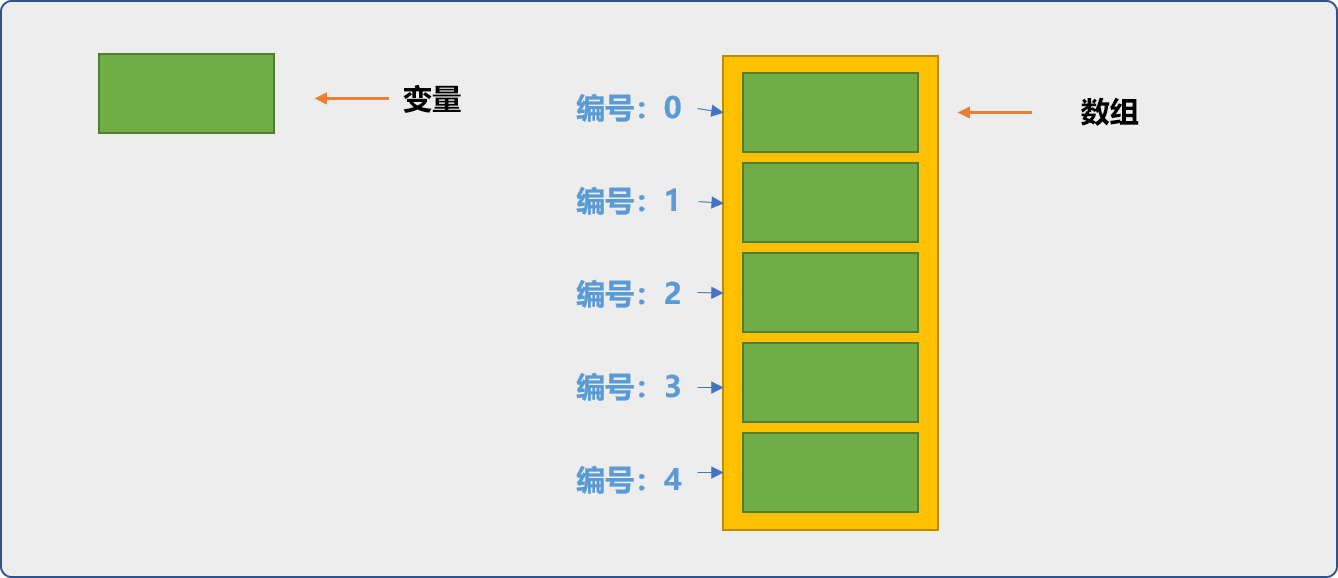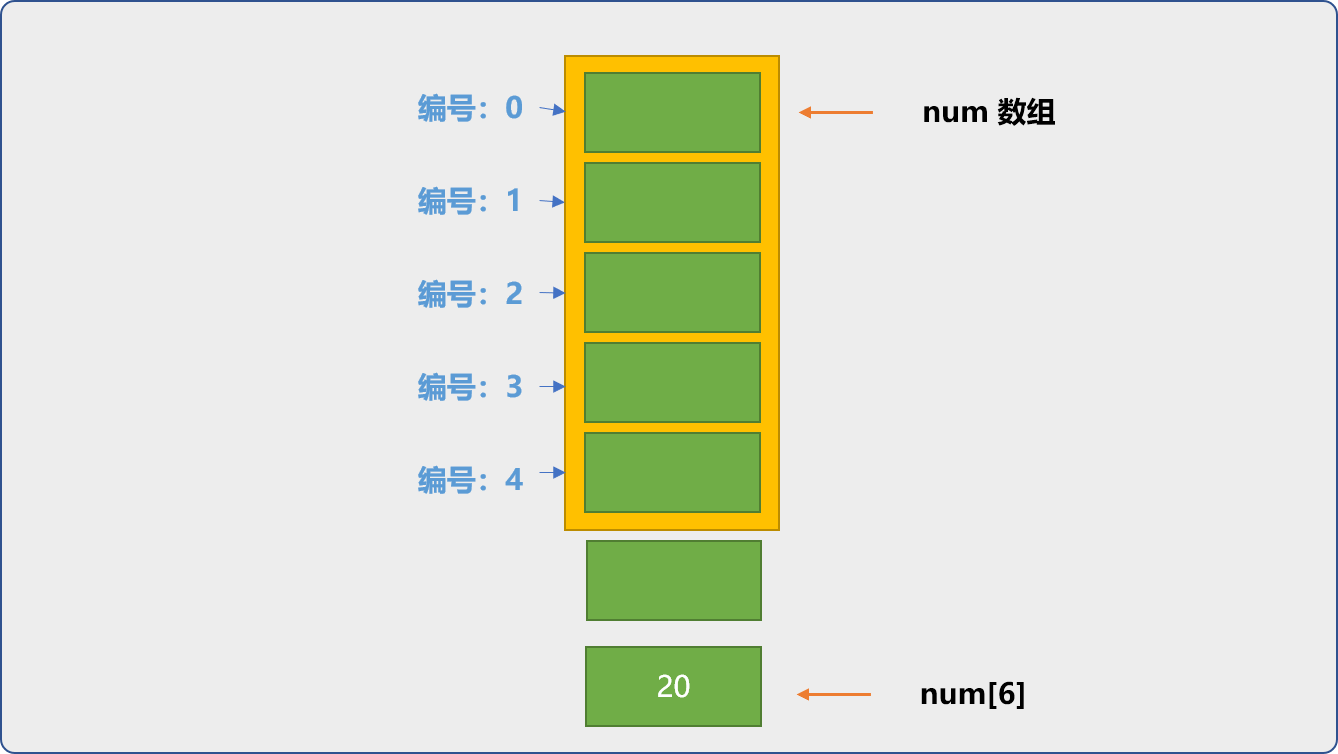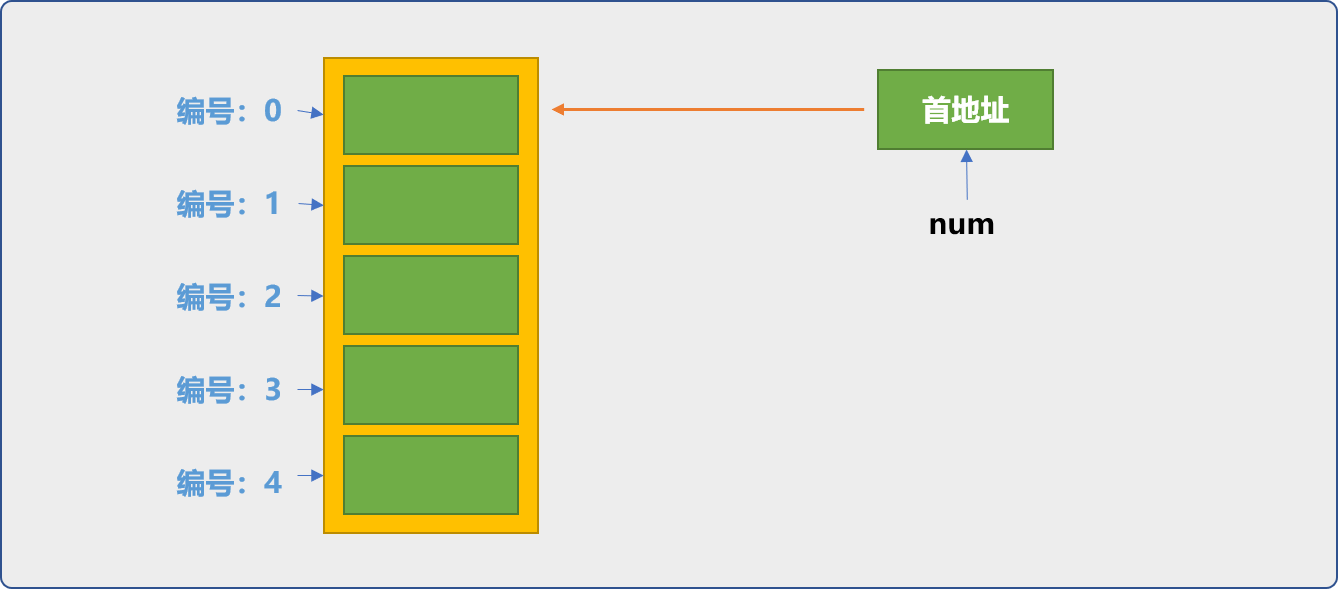## 1. 数组概念

• 同类型多个变量的集合。
• 每一个变量没有自己的名字。
• 数组会为每一个变量分配一个位置编号 。
• 可以通过变量在数组中的位置编号（下标）使用变量。

`C++`中称`数组`为复合类型，复合类型指除了基本类型之外或通过基本类型组合而成的新类型。如类、结构体、枚举……## 2. 创建数组

• 静态创建。
• 动态创建。

### 2.1 静态创建

#### 2.1.1 语法

• `数组名``数组名`就是`变量名`，只是这个名称指的是一片连续区域。
• `数据类型`：数组用来保存什么样类型的数据。
• `数组长度`：编译器需要根据数组大小开辟空间。
``````int num;
``````

``````int num;
cout<<num<<endl;
``````

``````int num;
for(int i=0;i<10;i++){
cout<<num[i]<<endl;
}
``````

``````1
0
4254409
0
0
0
34
0
0
0
``````

#### 2.1.2 初始化

• 创建后通过循环语法结构赋值。
``````int num;
for(int i=0; i<10; i++) {
num[i]=i*10;
}
``````
• 单个变量赋值。
``````int num;
num=10;
``````
• 边创建边赋值，`{}`符号可以用来表示数组字面常量。
``````//正确
int num={1,3,4,9};
``````

``````//错误
int num={1,3,4,9};
``````

``````int num;
//错误
num=={1,3,4,9};
``````

``````int num[]={1,3,4,9};
``````
• 全部初始化为 `0`。如下代码，初始化时只指定一个值且为 `0` 时，这里的语义不是指给数组中的第一个变量赋值，而是为数组中的所有变量指定初始值为 `0`
``````int num={0};
//输出数组所有值
for(int i=0; i<5; i++) {
cout<<num[i]<<endl;
}
``````

``````0
0
0
0
0
``````

``````int num={1,0};
for(int i=0; i<5; i++) {
cout<<num[i]<<endl;
}
``````

``````1
0
0
0
0
``````

``````int num={1,2,0};
for(int i=0; i<5; i++) {
cout<<num[i]<<endl;
}
``````
• `C++11`中提供更清晰、简洁、安全的初始化语法。如下语法，是不是很简洁、惊艳。数组和`{}`之间可以不用等于号，太体贴了，生怕你多敲一个字母，会手痛。且为数组中的每一个变量赋值 `0`。没有多余的废话。
``````int num {};
for(int i=0; i<5; i++) {
cout<<num[i]<<endl;
}
``````

``````int num= {};
``````

``````int num ={3,2.5};
``````

#### 2.1.3 越界问题

`C++`中使用数组，没有访问`越界`一说。所谓访问`越界`，指`下标`超过数组创建时指定的大小范围。

``````int num;
//理论是越界的
num=20;
for(int i=0; i<7; i++) {
//输出了 7 个数据
cout<<num[i]<<endl;
}
````````````0
0
34
0
0
0
20
``````

`C++`并不会阻止你的访问超过数组边界，但是，开发者需要从源头上切断这种行为。类似于相邻两家，关系很好，相互之间不设阻隔墙，但不意味着你能随意出入对方家里。

#### 2.2.4 数组与指针

• 存储数组数据的内存区域。
• 存储数组首地址的内存变量。`数组名`本质是指针变量，保存着数组的首地址，也是第一个存储位置。

``````int num={4,1,8,2,6};
cout<<"数组的地址："<<num<<endl;
//输出结果：
//数组的地址：0x70fe00 16进制描述的内存地址
``````

``````int num={4,1,8,2,6};
cout<<"数组中的第一个位置的数据："<<*num<<endl;
//数组中的第一个位置的数据：4
``````

``````int num={4,1,8,2,6};
cout<<"数组中的第一个位置的数据："<<num<<endl;
//数组中的第一个位置的数据：4
``````

Tip： `num+1`可以让指针移到数组的下一个变量位置。这里的`1`具体移动多少，由创建数组时指定的数据类型决定，如本文数组是 `int`类型，`1`便是移动 `4 字节`

``````int num={4,1,8,2,6};
cout<<"数组中第二个位置的数据："<<*(num+1)<<endl;
//数组中第二个位置的数据：1
``````

``````int num={4,1,8,2,6};
cout<<"数组中第二个位置的数据："<<num<<endl;
``````

``````int num={4,1,8,2,6};
//完全超界，但人家就是能运行 ，指针的手能伸到天涯海角
for(int i=0;i<1000000;i++){
cout<<*(num+i)<<endl;
}
``````

#### 2.2.5 小结

• `编译`时，就需要为`数组`指定大小，或说数组大小在编码时就必须给定。
• 静态创建数组时不能使用 `auto`关键字。
``````//错误语法
auto  num;
``````
• 数组名中保存有数组大小的信息。如下代码可以获取到数组长度。
``````int num;
//sizeof得到num实际占用的内存空间，以字节为单位
int len=sizeof(num)/4;
cout<<len;
//输出：10
``````
• 静态数组的数据保存在`栈`中，在编译期间进行空间分配，在生命周期结束后自动回收。

### 2.2 动态创建

• 开发者可以根据自己的需要提出空间使用申请。
• 当空间不再需要时，开发者需要手动释放空间。

``````int *num=new int;
``````

• `num`是指针变量，用来保存数组的首地址。
• `new`是运算符，其作用是在堆中开辟空间，并把空间的首地址返回。
• `int`，指开辟空间的大小，以及保存什么类型的数据。

`num`是首地址，也是数组中第一个位置的地址。

``````int *num=new int;
//初始化第一个位置的数据
*num=10;
cout<<"第一个位置的数据:"<<*num<<endl;
``````

``````int *num=new int;
for(int i=0; i<10; i++) {
*(num+i)=i*10;
}
for(int i=0; i<10; i++) {
cout<<*(num+i)<<endl;
}
``````

``````0
10
20
30
40
50
60
70
80
90
``````

``````int *num=new int;
for(int i=0; i<10; i++) {
num[i]=i*10;
}
for(int i=0; i<10; i++) {
cout<<num[i]<<endl;
}
``````

``````int *num=new int;
num=new int;
``````

``````int *num=new int;
cout<<sizeof(num)/4<<endl;
//输出结果：2，并不是数组的长度。
``````

``````int *num=new int;
//delete num;语法上可行，会产生不确定行为
delete [] num;
``````

## 3. 数组性能分析

``````//最后一位为预留位置
int num= {1,2,3,4,5,6,7,8,9,0};
for(int i=0; i<10; i++) {
cout<<num[i]<<"\t";
}
cout<<endl;
int newNum=0;
int pos=0;
cout<<"请输入要插入的数据："<<endl;
cin>>newNum;
cout<<"请输入要插入的位置："<<endl;
cin>>pos;
//从插入位置的数据向后移动
for(int i=9; i>pos-1; i--) {
num[i]=num[i-1];
}
num[pos-1]=newNum;
for(int i=0; i<10; i++) {
cout<<num[i]<<"\t";
}
``````

``````1       2       3       4       5       6       7       8       9       0

13

5
1       2       3       4       13      5       6       7       8       9
``````

``````int num= {1,2,3,4,5,6,7,8,9,10};
for(int i=0; i<10; i++) {
cout<<num[i]<<"\t";
}
cout<<endl;
int pos=0;
cout<<"请输入要删除的位置："<<endl;
cin>>pos;
//从插入位置的数据向前移动
for(int i=pos-1; pos<8; i++) {
num[i]=num[i+1];
}
//最后一位补 0
num=0;
for(int i=0; i<10; i++) {
cout<<num[i]<<"\t";
}
``````

``````1       2       3       4       5       6       7       8       9       10

4
1       2       3       5       6       7       8       9       10      0
``````